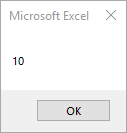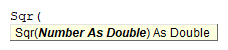# VBA Sqr Function

## Sqr Function

Returns the square root of a number.

## Simple Sqr Examples

``````Sub Sqr_Example()
MsgBox Sqr(100)
End Sub
``````

This will return 10## Sqr Syntax

In the VBA Editor, you can type  “Sqr(” to see the syntax for the Sqr Function:The Sqr function contains an argument:

Number: Any valid numeric expression greater than zero.

## Examples of Excel VBA Sqr Function

``MsgBox Round(0.25)``

Result: 0.5

``MsgBox Round(25)``

Result: 5

``MsgBox Round(-25)``

Result: Run-time error ‘5’

Stop searching for VBA code online. Learn more about AutoMacro - A VBA Code Builder that allows beginners to code procedures from scratch with minimal coding knowledge and with many time-saving features for all users!